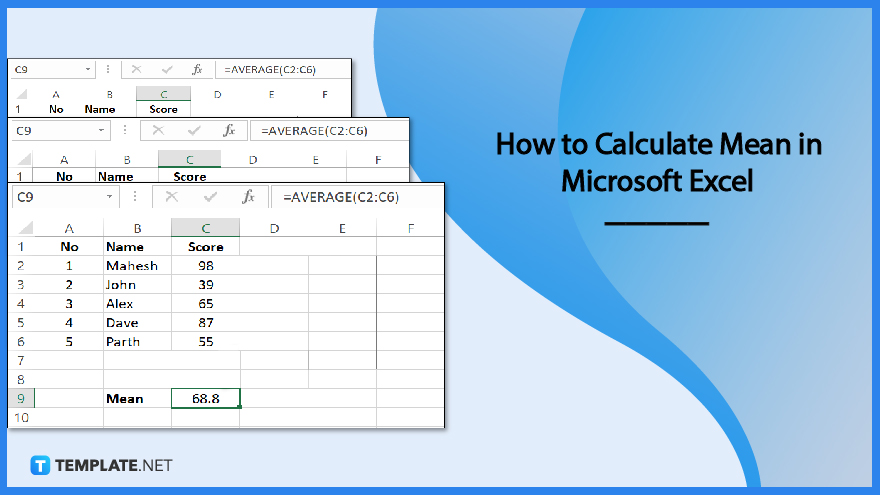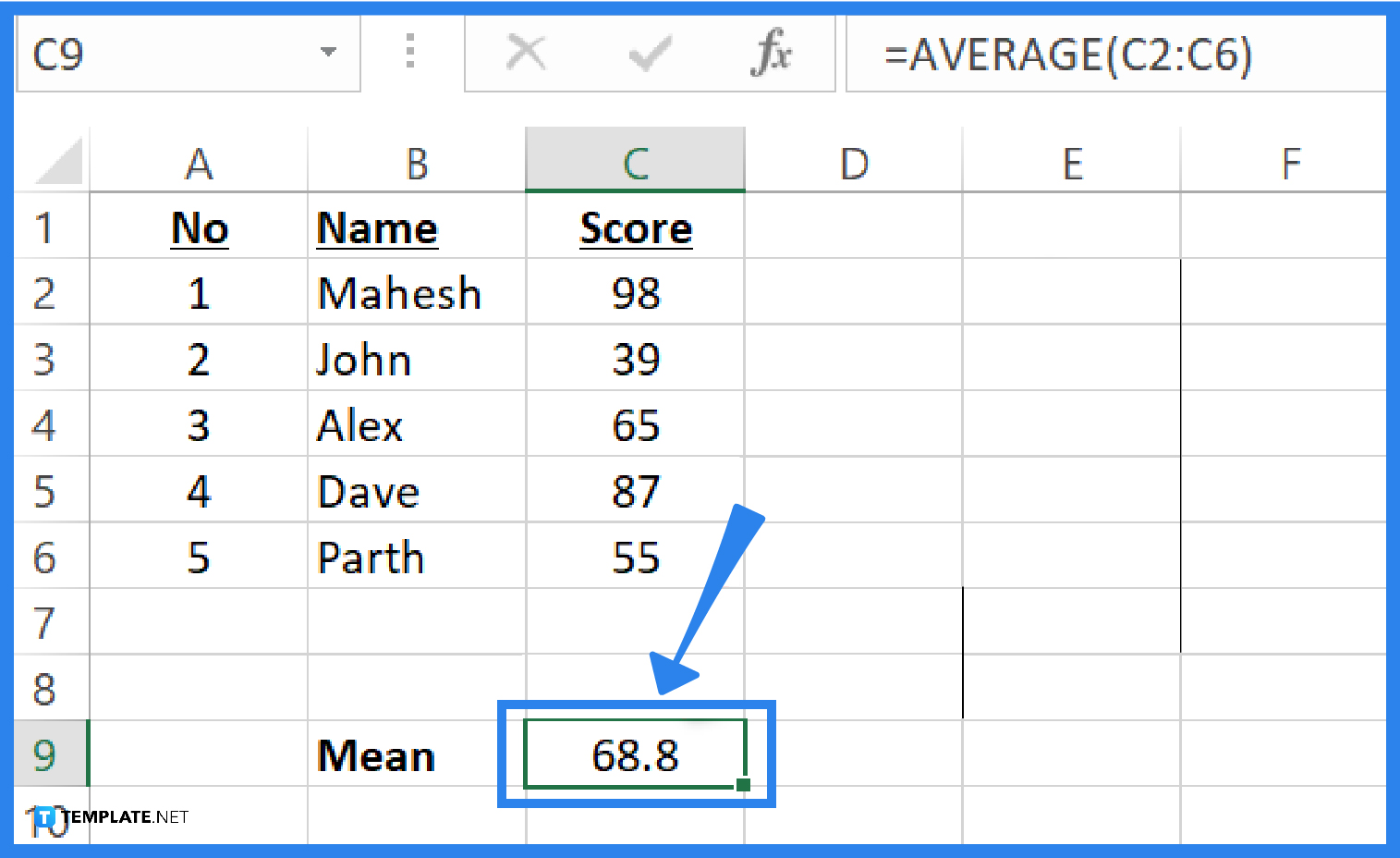# How to Calculate Mean in Microsoft Excel

Every type of data processing and analysis benefits from knowing how to find the mean. Using the AVERAGE function in Microsoft Excel, you can quickly and easily calculate the mean for your variables.## How to Calculate Mean in Microsoft Excel

The sum of all the values in the set divided by the number of those values is, by definition, the mean for a data set. The AVERAGE function in Microsoft Excel makes it simple to calculate the mean.

• ### Step 1: Launch Microsoft Excel

To launch Microsoft Excel, you can go to the Start icon on your desktop and locate Microsoft Excel. You can also search for it in the “Search” bar of your desktop to find it fast, or you can also locate it in the dock of your desktop. Just click it to launch.

• ### Step 2: Input the Variables

The sum of all the numbers in the data set is used to compute the mean of the data set, which is then divided by the total number of values in the data set. Start by entering some data into your spreadsheet. Some examples that you can input as a data set are salary, score, age, or anything that involves numbers. You can also use some existing data or variables that you already have.

• ### Step 3: Calculate the Mean• Click on the cell where you want to put the mean of your variables. Type in the formula for the mean using the AVERAGE function. The syntax is =AVERAGE(array of variables). For example, if you want to find the mean for the values in all the cells from C2 to C6, then your formula should be written like this: =AVERAGE(C2:C6). After that, hit the Enter button and the resulting mean will show up in the cell you selected.

## FAQs

### What distinguishes the mean from the average in Microsoft Excel?

The words “average” and “mean” are frequently used interchangeably. The average is derived from sets of numbers that are almost identical, meaning that there is little variation between them. While the mean is derived for sets of values that differ significantly from one another or are closely related. However, the term “mean” is used in statistics in place of the term “average”.

### What is another way of calculating the mean in Microsoft Excel?

In this procedure, you must first choose the cells for which the mean must be calculated. A dialog box will then display once you choose the INSERT function from the formulas tab. Enter the cell range for your list of numbers in the “Number1” field after selecting the AVERAGE function from the dialog. For instance, use A1:A10 if you require values from column A and rows 1 through 10. You can alternatively just click OK after dragging and dropping the chosen cells. The cell you’ve chosen will display the outcome for the mean.

### What are AVERAGEIF and AVERAGEIFS for calculating the mean in Microsoft Excel?

The AVERAGEIF function is used to calculate the average of only the values that satisfy a single condition, but the AVERAGEIFS function is used to calculate the average of values based on many conditions.